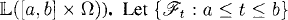# MARTINGALE STOCHASTIC INTEGRALS

In the previous two chapters, we introduced stochastic integrals with respect to standard Brownian motions. In this chapter, we expand the definition of stochastic integral such that the integrators are right-continuous and square-integrable martingales.

# 34.1 Basic Concepts and Facts

Definition 34.1 (Stochastic Process Spacebe a right-continuous filtration. The stochastic process space([a, b] × Ω) is the space of all stochastic processes X(t, ω) satisfying the following conditions:

(a) X(t) is adapted to the filtration.
(b) Almost all sample paths of Xt are left-continuous.

Definition 34.2 (Predictable Stochastic Process). Letbe the smallest σ-field of subsets of [a, b] × Ω with respect to which all stochastic processes in([a, b] × Ω) are measurable. A stochastic process {Xt : atb} is said to be predictable if the functionis -measurable. ...

Get Measure, Probability, and Mathematical Finance: A Problem-Oriented Approach now with the O’Reilly learning platform.

O’Reilly members experience live online training, plus books, videos, and digital content from nearly 200 publishers.